# The library

Anna stored a total of 126 books in three compartments of the library. There are 6 books more in the first compartment than in the second one. The number of books in the second compartment is the arithmetic average of the number of books in the first and third compartments. Calculate how many books are in each compartment?

a =  48
b =  42
c =  36

### Step-by-step explanation:

a+b+c=126
a = 6+b
b = (a+c)/2

a+b+c = 126
a-b = 6
a-2b+c = 0

a = 48
b = 42
c = 36

Our linear equations calculator calculates it.Did you find an error or inaccuracy? Feel free to write us. Thank you!Tips to related online calculators
Looking for help with calculating arithmetic mean?
Looking for a statistical calculator?
Do you have a system of equations and looking for calculator system of linear equations?

#### You need to know the following knowledge to solve this word math problem:

We encourage you to watch this tutorial video on this math problem:

## Related math problems and questions:

• In the libraryIn the library at Lenape Elementary School, there are 2/5 as many fiction books as there are nonfiction books. There are 56 books in the school library. How many books are fiction books?
• Stamps and albumsMichail has in three albums stored 170 stamps. In the first album, there are 14 more than in the second and in the second is by 1/5 less than in the third. How many stamps are in the first album?
• Three workshopsThere are 2743 people working in three workshops. In the second workshop works 140 people more than in the first and in third works 4.2 times more than the second one. How many people work in each workshop?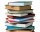Readers borrowed a total of 220 books in the library during the first three days. On the second day, readers borrowed half as many books as the first day and at the same time 20 fewer books than the third day. Depending on the quantity x, express the numb
• The HotelThe Holiday Hotel has the same number of rooms on each floor. Rooms are numbered with natural numerals sequentially from the first floor, no number is omitted, and each room has a different number. Three tourists arrived at the hotel. The first one was in
• 925 USDFour classmates saved an annual total 925 USD. The second save twice as the first, third 35 USD more than the second and fourth 10 USD less than the first. How USD save each of them?
• BoxesTwo hundred boxes have been straightened in three rows. The first row was 13 more than in the second, and in the second was one fifth more than in the third one. How many boxes are in each row?
• The libraryThere are 65 boxes of books. Each box contains 100 books. If each book costs 19.75 how much is the total cost of all the books?
• Three unknown numbers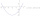The sum of of three numbers is 106. the first number is 10 more then the third. The second number is 2x the third. What are the numbers?
• SchoolsThree schools are attended by 678 pupils. To the first attend 21 students more and to the third 108 fewer students than to second school. How many students attend the schools?
• Employees1116 people are working in three factory halls. In the first one, there are 18% more than the third, and 60 persons more than the second. How many employees work in individual halls?
• MilkThere were 22 liters of milk in three containers. There was 6 liters more in the first container than in the second. After pouring 5 liters from the first container into the third container, the same quantity of milk is in the second and third container.
• RepairingThree employees earned a total of € 469 for repairing the equipment. They split so that the first got 20% more than the second, and the third 15% more than the second. How many euros did everyone get?
• Three granaries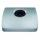Three granaries hold 7,800 metric cent (q) of grain. In the first, 70 tonnes of grain was more than the second and in the third it was 120 tonnes less than in the second. How many grain were in each granary?
• The farm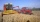The farm harvested 390 tons of grain. Wheat was 15% more than barley, and rye was 126 tons less than wheat and barley combined. How many tons of wheat, how many tons of barley, and how much rye did the team get?
• The nuts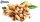There are 159 nuts in two bags, 15 more in the first than in the second, how many nuts are in the bags?
• Potatoes bags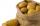I have three bags with 21 kg potatoes. The first bag is 5.5 kg more than in the second bag, and the third is 0.5 kg more than in the second bag. Determine how many kgs of potatoes are in each bag.